Journal of Vibroengineering

Published: 15 December 2020

# Unsteady aerodynamic identification based on recurrent neural networks

Bo Zhang1
Jinglong Han2
Tuanyuan Zhang3
Ruiqun Ma4
1, 2, 4State Key Laboratory of Mechanics and Control of Mechanical Structures, Nanjing University of Aeronautics and Astronautics, Nanjing, 210016, China
3China Academy of Aerospace Aerodynamics, Beijing, 100074, China
Corresponding Author:
Jinglong Han
Views 193

#### Abstract

The dynamic stall at high angle of attack is an important aerodynamic problem faced by aircraft, and it has always been a hotspot of aerodynamic research. The traditional reduced order model (ROM) methods needs to establish an accurate model, and has a high demand for experience. In this paper, a novel nonlinear aerodynamic identification method based on recurrent neural networks (RNNs) is proposed. The computational fluid dynamics (CFD) method is used to calculate the unsteady aerodynamic parameters of the NACA0012 airfoil. A group of sinusoidal chirp signals with variable amplitude and frequency are adopted as the excitation signals, and the obtained data are used to train the recurrent neural networks, and the ROM of the nonlinear aerodynamic model of high angle of attack dynamic stall is obtained. Finally, the aerodynamic parameters of a group of composite sinusoidal motion signals different from the training signals are predicted by the trained neural networks model and compared with the CFD results.

## 1. Introduction

Dynamic stall refers to the unsteady separation of the flow field around an oscillating or other unsteady airfoils when it exceeds the critical angle of attack, which leads to stall. For example, a dynamic stall occurs in the flow of a fighter’s post-stall maneuver, helicopter blade rotation, aeroengine’s turbine and fan blade flutter. Its main feature is the existence of complex unsteady separation vortex and large-scale structure vortex in the flow field, the vortex turbulence separates from the leading edge or trailing edge area of the airfoil, and the airfoil keeps stalling until the air flow re-adheres to the airfoil. Compared with the static stall, the dynamic stall is more complex. The lift, moment and drag characteristics of the airfoil vary with the different motion forms of the airfoil, and there will be obvious nonlinear hysteresis phenomenon at the maximum angle of attack when the dynamic stall occurs. Once dynamic stall occurs, the consequences are more serious and the duration is longer . The aerodynamic coefficient may greatly deviate from the static value. So the simple static stall research can’t meet the needs of dynamic stall research.

At present, the research of dynamic stall mainly adopts numerical simulation or wind tunnel test methods. Wind tunnel test can measure the aerodynamic force and pressure of the reduced ratio airfoil in the wind tunnel, which can intuitively observe the hysteresis effect of aerodynamic force in the process of dynamic stall of airfoil. However, the wind tunnel test method is difficult to capture the generation, separation and reattachment of vortices in the oscillating process of airfoil, its preliminary preparation and specific wind tunnel test process are very complex, expensive and time-consuming, which can only be used for principle research of specific working conditions. The numerical simulation method can overcome the shortcomings and difficulties of the wind tunnel test . It can simulate the dynamic stall of airfoil under various conditions and capture the whole falling off process of the detached vortices. This method has become an important way to study the unsteady dynamic stall characteristics.

Numerical methods can be divided into semi empirical model based on test data and computational fluid dynamics (CFD) method. Researchers have developed a series of semi empirical dynamic stall theoretical models, such as Gangwani model , ONERA model , Leishman-Beddoes (L-B) model . The Gangwani model is the early semi empirical model. The ONERA model and Leishman Beddoes (L-B) model are internationally recognized as two-dimensional aerodynamic force and widely used. Although the above two methods have achieved the engineering application accuracy and greatly reduced the calculation time, the semi empirical model is not a strict prediction method, which is only applicable to specific airfoil, and there is a big deviation between the calculated value and the test value of the peak value in the whole stall process and the aerodynamic force during the reattachment after the flow separation. In addition, the semi empirical model can only calculate the aerodynamic force effectively, but it can't simulate the details of the vortex flow in the complex separation and reattachment phenomenon in the unsteady flow field of the airfoil. It’s difficult to carry out further research for semi empirical model due to the above reasons. The computational fluid dynamics method can capture the detail of the formation, development, shedding and reattachment process of the vortex in the dynamic stall process, which is an important means to study the dynamic stall problem. Dubuc  used the implicit dual-time step method to solve the Euler equation to study the unsteady motion of a NACA0012 airfoil with small amplitude transonic oscillation and accurately simulate the hysteresis effect of the airfoil in the shallow stall state. Later, a grid deformation algorithm based on transfinite interpolation method was developed and verified by the forced oscillation numerical simulation of flap validity of the method .

The CFD method has a high calculation accuracy, but it needs a lot of time and computing resources. Therefore, the reduced order model (ROM) methods for complex nonlinear systems have been widely concerned, such as proper orthogonal decomposition (POD) , Volterra series , dynamic mode decomposition (DMD) [10, 11], etc. However, the shortcomings of these methods in nonlinear and multi-scale simulation limit their application. Lucia [12, 13] developed a kind of unsteady aerodynamic reduced order model based on CFD technology, which was successfully used to study the flutter and gust response dynamics of aircraft at transonic speed or high angle of attack. However, most of these ROM methods are dynamic linear models, which could take into account the static nonlinear characteristics of the flow at transonic speed or high angle of attack, but cannot be used for the aerodynamic modeling research with large amplitude shock motion, unsteady separation and other dynamic nonlinear characteristics.

The traditional ROM methods has a high demand for experience, which is difficult to give a suitable explicit expression due to the complexity of the nonlinear flow field. The neural networks can deal with high-dimensional problems and does not need to give the explicit mathematical expression between the input and output of the identification system, which is an ideal tool for nonlinear aerodynamic prediction. Marques  identified the unsteady aerodynamic forces of transonic airfoils using an artificial neural networks with multi-layer functions. Zhang [15, 16] proposed a nonlinear unsteady aerodynamic layered reduced order model based on radial basis function networks (RBFNN), and used the model to predict the unsteady aerodynamic force, limit cycle oscillation and flutter characteristics of AGARD wing 115.6 and NACA 64A010 airfoils. Winter  proposed a nonlinear aerodynamic reduction scheme based on the series connection of a recurrent local linear neural fuzzy model and multilayer perceptron neural networks. Wang  uses a long-short term memory (LSTM) neural networks to construct a set of hypersurfaces representing the reduced fluid dynamic system. Afshar  proposes a flow field prediction model based on convolutional neural networks (CNNs), which is successful to predict the velocity and pressure field in geometry given the pixelated shape of the object and unseen flow conditions. Mannarino  develops a reduced order method based on continuous time recurrent neural networks (CTRNN) identification. Their researches show that deep neural networks identification is efficient for dealing with fluid dynamic problems with strong nonlinearity.

In this paper, a novel reduced order model method based on recurrent neural networks is presented to predict the nonlinear unsteady aerodynamic forces at high angle of attack. The unsteady aerodynamic force of NACA0012 airfoil at deep stall is calculated by the CFD method. The results are compared with the experimental data, and the effectiveness of the CFD model is verified. Two kinds of unsteady aerodynamic forces are calculated by CFD method: a variable frequency and amplitude motion is used as the identification training input signals; a composite sine signal is used to verify the effectiveness of the identification system. The trained recurrent neural networks is used for unsteady aerodynamic force identification, and the results predicted by neural networks are compared with the results of CFD.

## 2. Recurrent neural networks

### 2.1. Networks structure

Artificial neural networks can be divided into forward neural networks (FNNs) and recurrent neural networks (RNNs) according to whether there is feedback nodes. The architecture of basic forward neural networks is shown in Fig. 1.

Fig. 1Architecture of forward neural networks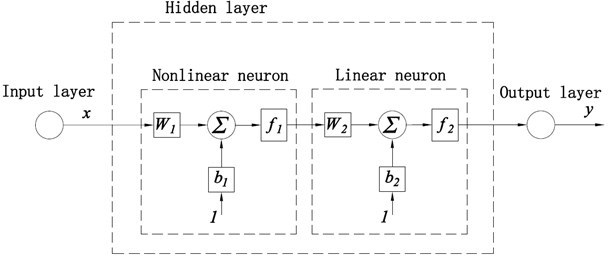There is not delay layer and feedback nodes in the FNNs, which cannot deal with the time series problems and dynamic problems. Its mapping relationship between input and output can be expressed as:

1
$y=f\left(x\right)={f}_{2}\left({W}_{2}{f}_{1}\left({W}_{1}x+{b}_{1}\right)+{b}_{2}\right),$

where $y$ is the output of the system; $f$ represents the transfer function of the system; $x$ is the input of the system; ${f}_{1}$ and ${f}_{2}$ denote the transfer functions of the neuron, respectively; ${W}_{1}$ and ${W}_{2}$ represent the weight of the neuron, respectively; and ${b}_{1}$ and ${b}_{2}$ are the biase of the neuron, respectively.

The structure of recurrent neural networks is shown in the Fig. 2.

Its mapping relationship between input and output can be expressed as:

2
$y\left(t\right)=f\left(y\left(t-1\right),y\left(t-2\right),\dots ,y\left(t-p\right),x\left(t-1\right),x\left(t-2\right),\dots ,x\left(t-q\right)\right),$

where $y\left(t\right)$ denotes the output of the system at $t$ moment; and $x\left(t\right)$ represents the input of the system at $t$ moment.

### 2.2. Optimization algorithm

Fig. 2Architecture of recurrent neural networks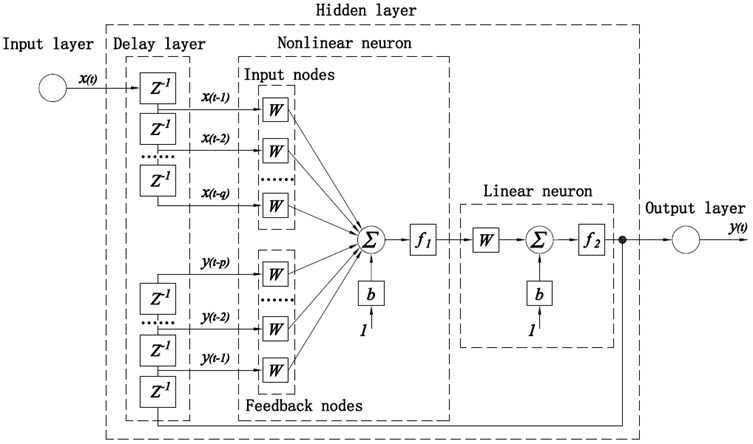The L-M method is an iterative technique, which can find the minimum value of multivariate function expressed as the sum-of-squares of nonlinear real valued functions :

3
$E\left(x\right)=\sum _{i=1}^{m}{F}_{i}^{2}\left(x\right),$

where $E\left(x\right)$ is the minimum value of multivariate function, $i$ is the training pattern index and ${F}_{i}\left(x\right)$ is the error for pattern $i$, which can be defined by the following formula:

4
$F\left(x\right)=\left[\begin{array}{c}y\left(x,{t}_{1}\right)-\phi \left({t}_{1}\right)\\ y\left(x,{t}_{2}\right)-\phi \left({t}_{2}\right)\\ \begin{array}{c}\cdots \\ y\left(x,{t}_{m}\right)-\phi \left({t}_{m}\right)\end{array}\end{array}\right],$

where $y\left(x,{t}_{i}\right)$ and $\phi \left({t}_{i}\right)$ are scalar functions.

The weights of RNNs are updated by the following solutions of linear equations:

5
${d}_{k}=-{\left[J{\left({x}_{k}\right)}^{T}J\left({x}_{k}\right)+{\lambda }_{k}I\right]}^{-1}J{\left({x}_{k}\right)}^{T}F\left({x}_{k}\right),$

where $J\left({x}_{k}\right)$ is the Jacobian matric of $F\left({x}_{k}\right)$; ${\lambda }_{k}$ is a scalar regulation which controls the modulus sum direction of ${d}_{k}$ and $I$ is the identity matrix. When ${\lambda }_{k}$ is zero, the direction ${d}_{k}$ is the same as the Newton method. As ${\lambda }_{k}$ tends to infinity, ${d}_{k}$ tends to the steepest descent direction and the mode tends to zero, which means that when ${\lambda }_{k}$ is large enough, the term $F\left({x}_{k}+{d}_{k}\right) holds. Therefore, the term ${\lambda }_{k}$ can be controlled to ensure the descent even if the second-order term which will limit the efficiency of the Newton method is encountered. Hence, the search direction of L-M method combines the advantages of Newton direction and steepest descent direction.

## 3. CFD simulation of aerodynamic at high angle of attack

### 3.1. Grid model

The spring grid method and transfinite interpolation method, which are widely used at present, can effectively avoid the appearance of negative volume and grid distortion, and have certain accuracy for small amplitude oscillation motion. However, it is difficult to ensure the grid orthogonality and smoothness when the airfoil with large deformation and large amplitude oscillation motion, which will result in grid distortion and negative volume and cannot be calculated. The nested grid allows the grid in different regions to perform independent parallel computing without changing the grid shape, and coupling by interpolation. Therefore, nested grid technology is often used to study the dynamic stall of high angle of attack. This work adopt the nested grid.

In this paper, the ICEM is used to generate the computational grid. Firstly, the body fitted grid around the airfoil is generated, which is a C-H type foreground structure grid with the boundary condition set to overset. Then, the fixed H type background structure grid is generated. The two types of grid are merged by interpolation. The distance between the boundary of the background grid and the airfoil is 15 times of the chord length. The thickness of the first layer grid on the airfoil surface is 0.001 mm, and the growth rate of grid thickness is 1.1. The total number of elements is 365482. Fig. 3 shows the structure of the nested grid and the boundary condition setting. From the area around the airfoil surface, it can be seen that the grid has better performance of orthogonality and smoothness, which provide a good basis for the Reynolds averaged Navier-Stokes (N-S) equation to capture the viscous and high angle of attack flow separation. The user-defined functions (UDF) is used to define the region motion of the foreground grid in the nested grid, which is used to control the motion of the airfoil.

Fig. 3Nested mesh and local enlargement diagram of NACA0012 airfoil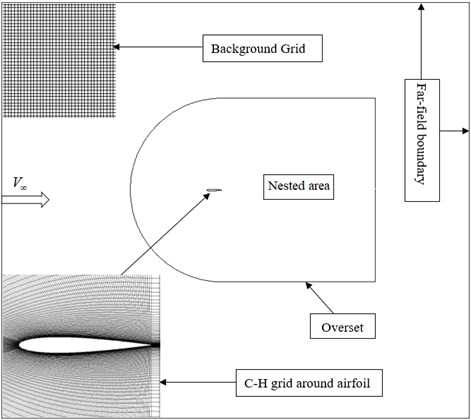### 3.2. Numerical simulation of unsteady dynamic stall of airfoil

In this section, the CFD model of NACA0012 airfoil is established, nested grid is employed to divide flow field, Transition SST $k–\omega$ model is chosen as the viscous model. According to the experiments in reference , the airfoil makes large amplitude oscillation around 1/4 chord length point. The move equation is shown as bellow:

6
$\alpha ={\alpha }_{0}+{\alpha }_{1}\mathrm{sin}\left(k\tau \right),$

where ${\alpha }_{0}$ is the average angle of attack; ${\alpha }_{1}$ is the amplitude angle of attack; $k$ is the reduction frequency; and $\tau$ is dimensionless time (reduction time). $k$ and $\tau$ can be defined as follows :

7
$k=\frac{\pi fc}{{u}_{\mathrm{\infty }}},$
8
$\tau =\frac{2{u}_{\mathrm{\infty }}t}{c},$

where ${u}_{\infty }$ is the flow velocity in the far field; $t$ is the time; $f$ is the oscillation frequency; and $c$ is the reference chord length of the airfoil. The Reynolds number based on the chord length is defined by the following formula :

9
$Re=\frac{\rho {u}_{\infty }c}{\mu },$

where $\rho$ is the density of inflow air; and $\mu$ is dynamic viscosity.

The above method is used to simulate the unsteady aerodynamic force of NACA0012 low speed large amplitude oscillation. The calculation conditions are shown in Table 1. The test values of this state are given in reference . Other calculation conditions are: solver type is density-based; pressure far-field boundary condition is adopted; the boundary of the overlapping region is set to overset; and the wall condition is slip free boundary. Firstly, the steady flow field of airfoil at the average angle of attack is calculated; then, set the calculated value of the steady flow field as the initial value of the unsteady calculation to ensure the accuracy of the transient calculation results. The calculation results are shown in Fig. 4.

Fig. 4CFD results compared with experiments: a) lift coefficient, b) moment coefficient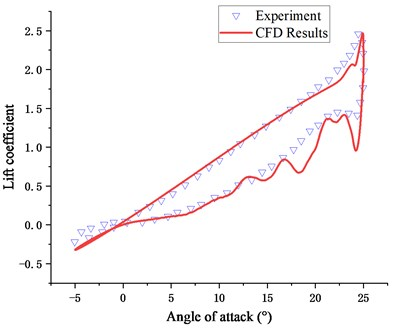a)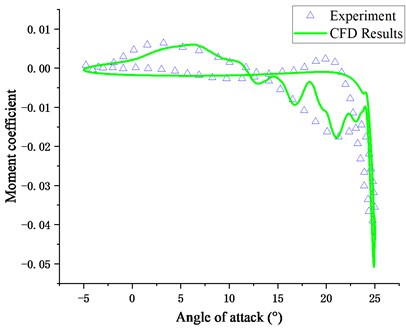b)

Fig. 4 shows the variation law of lift coefficient and moment coefficient in the process of airfoil motion. It can be seen from the figure that both lift coefficient and moment coefficient are basically the same as the experiment values . At the same time, the results of CFD can reflect the secondary stall which is not reflected in the test results. The results indicate that the calculation grid and numerical method adopted in this paper can prediction the nonlinear unsteady aerodynamic of airfoil at large angle of attack.

Table 1Nonlinear aeroelastic system parameters

 Parameters Definition Value ${u}_{\infty }$ Far field velocity 14 m/s $c$ Reference chord 1 $k$ Reduction frequency 0.1 ${\alpha }_{0}$ Average angle of attack 10° ${\alpha }_{1}$ Amplitude angle of attack 15° ${R}_{e}$ Reynolds number 1.35×105 $\rho$ Density of air 1.226 kg/m3 $T$ Temperature 288.15 K $∆t$ Time step 0.001 s

## 4. Results and discussion

### 4.1. Training data preparation

A group of sinusoidal chirp signals with variable amplitude and frequency are used as input signals for neural networks training. The maximum amplitude of pitch angle is 15°, and the frequency range is 0-3 Hz. The variation law of pitch angle is as follows:

10
${\alpha }_{1}=\left(0.0222{t}^{2}-0.667t+15\right)\mathrm{sin}\left(0.314{t}^{2}\right).$

This signal is used to establish the motion input of the airfoil, and the unsteady aerodynamic force of the airfoil under this motion is calculated by the CFD method as the training parameter of the neural networks. The trained RNNs is used to predict the lift and moment coefficient of the airfoil. The identification results of neural networks are shown in Fig. 5 and Fig. 6.

Fig. 5Lift coefficient identification results of chirp input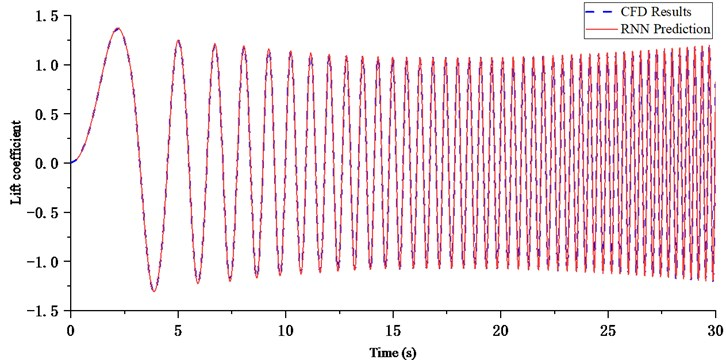The dotted line shows the response of computational fluid dynamics simulation, and the solid line shows the results of recurrent neural networks prediction. The two results are agreement very good, and it is hard to distinguish one from the other, which means that the trained networks could be used to predict the force of the unsteady aerodynamic.

### 4.2. Model verification

In order to verify the effectiveness of the identification model, a new set of composite sinusoidal motion signals, which are different from the training signals, are used as the verification input signals:

11
${\alpha }_{2}=5\mathrm{sin}\left(2\pi t\right)+3\mathrm{sin}\left(3\pi t\right)+2\mathrm{sin}\left(5\pi t\right).$

Fig. 6Moment coefficient identification results of chirp input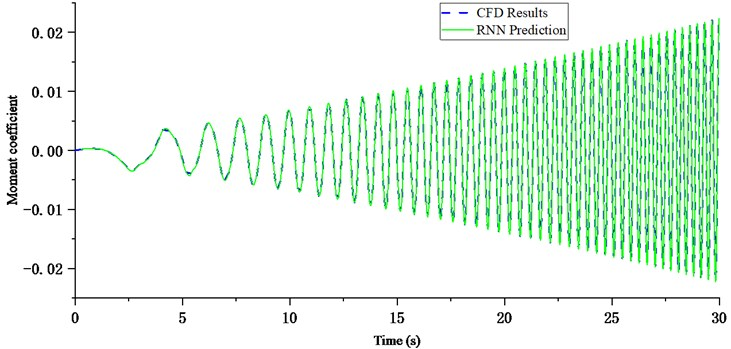Its maximum amplitude is 10°. Then, the trained neural networks model is used to predict the aerodynamic force of the airfoil in this motion. The predicted results are compared with the CFD results. The results are shown in Fig. 7 and Fig. 8.

Fig. 7Lift coefficient prediction results of verification signal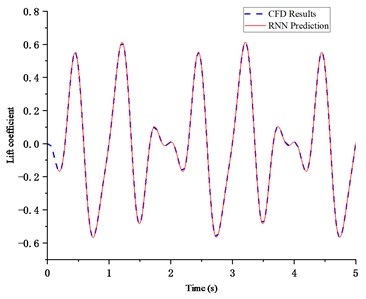Fig. 8Moment coefficient prediction results of verification signal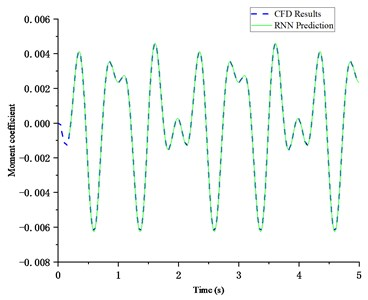The dotted line represents the CFD simulation results, and the solid line represents the RNNs prediction results. It can be seen from the figures that the two results are consistent, which indicates that the neural networks identification method can approach the nonlinear aerodynamic system very well.

The mean squared error (MSE) of the prediction data is used to evaluate the performance of the RNNs, which is defined as:

12
$MSE=\frac{\sum _{i=1}^{N}{\left(\stackrel{^}{y}\left(i\right)-y\left(i\right)\right)}^{2}}{N},$

where $\stackrel{^}{y}\left(i\right)$ is the prediction results of the RNNs; $y\left(i\right)$ represent the CFD results; and $N$ is the number of calculated dates. The MSE of the lift coefficient prediction is 4.0217×10-7, and the MSE of the lift coefficient prediction is 7.5282×10-10. The results show that RNNs can accurately predict the lift coefficient and moment coefficient, but the prediction of moment coefficient is more accurate.

## 5. Conclusions

In this work, the aerodynamic parameters of NACA0012 airfoil with sinusoidal motion around its 1/4 chord length are calculated by nested grid method, and compared with the test results, which verify the effectiveness of CFD method. A recurrent neural networks with time-delay element is used to approach the nonlinear aerodynamic system of large amplitude oscillation airfoil. A group of aerodynamic force of chirp motion with variable amplitude and frequency are obtained from the CFD method. The parameters of motion and aerodynamic force are used as input and output signals of the neural networks training. The trained networks is used to predict the aerodynamic force of composite sinusoidal motion. The results show that the neural networks ROM can accurately identify the nonlinear unsteady aerodynamic force of airfoil with large amplitude motion.

#### Acknowledgements

This work was supported by National Natural Science Foundation of China (Grant No. 11472133).

#### Cited by

Stall flutter prediction based on multi-layer GRU neural network
Aerodynamic Analyses of Airfoils Using Machine Learning as an Alternative to RANS Simulation